## Wednesday, 22 May 2013

### A number theory problem from online games Part 2

Using the same notation as last time we want to show that for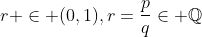,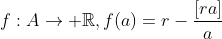(where A is natrual number larger than 1) maximized at the second crossings. Why?

Definition. Let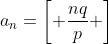be the largest integer that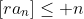.

The above claim is equivalent to claiming that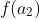is the global maximum of f.

Theorem.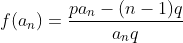.

Proof.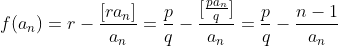, the result follows.

Theorem.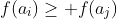iff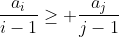.

Proof. Straightforward by direct expansion.

Theorem.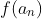maximized at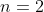.

Proof. This is equivalent to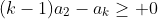.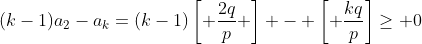which can be shown using modular arithmetic. (It isn't hard to check: there are only finite case to check.)

How about the case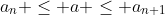?

Note that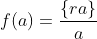and let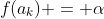. Consider the case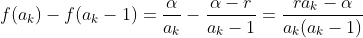which is larger than zero for large enough a_k. Now we can summarize our approach to find our optimal solution:

Check the following value of a: if they are a better solution replace the current one.
1) The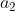(usually the best answer)
2) Find smallest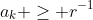. For each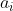find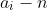so that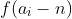is maximized in the range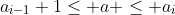.

Complexity? Something like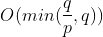. The problem is how often the answer falls onand how should we make the algorithm more efficient? I would leave that part open.